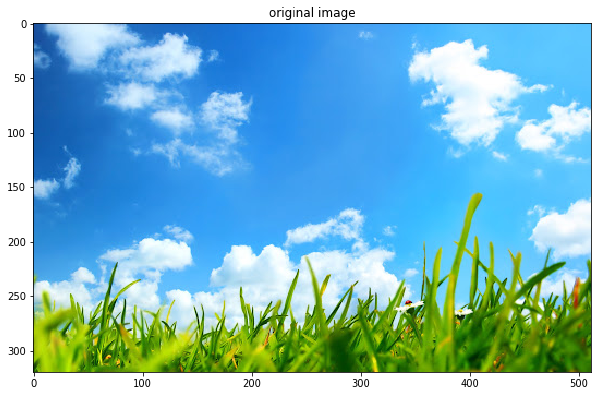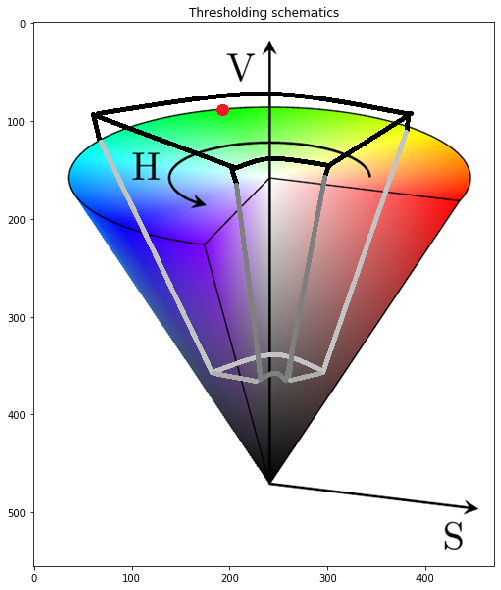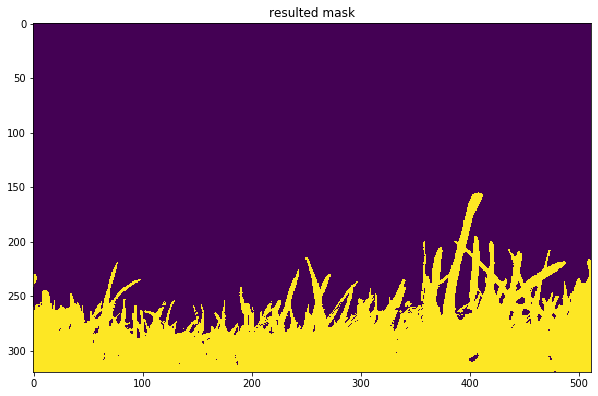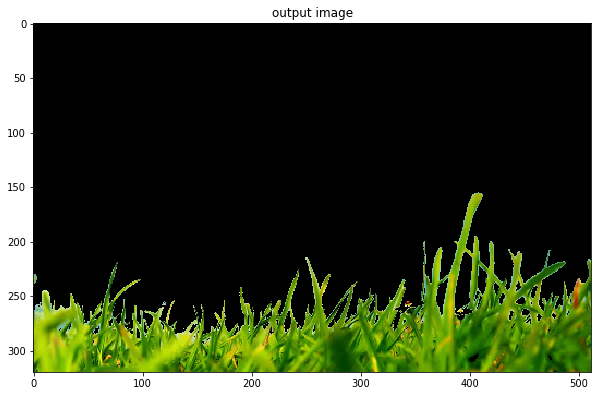In :
# to run in google colab
import numpy as np
import cv2
import matplotlib.pyplot as plt
import sys
import subprocess
subprocess.call('apt-get install subversion'.split())
subprocess.call('svn export https://github.com/YoniChechik/AI_is_Math/trunk/c_02a_basic_image_processing/grass.jpg'.split())

In :
# Adopted from: https://opencv-python-tutroals.readthedocs.io/en/latest/py_tutorials/py_imgproc/py_colorspaces/py_colorspaces.html

figsize = (10, 10)


In :
bgr_im = cv2.imread("grass.jpg")
rgb_im = cv2.cvtColor(bgr_im, cv2.COLOR_BGR2RGB)
plt.figure(figsize=figsize)
plt.imshow(rgb_im)
plt.title("original image")

Out:
Text(0.5, 1.0, 'original image')We want to separate the grass from the sky. we'll do this by masking all pixels in the image that are not green.

First, find HSV green

In :
rgb_green = np.uint8([[[0, 255, 0]]])  # 3d array just because this is what cvtColor expects...
hsv_green = cv2.cvtColor(rgb_green, cv2.COLOR_RGB2HSV)[0, 0, :]
print(hsv_green)

[ 60 255 255]


Second, convert the image to HSV and threshold only the green color and neighborhood.

We will take hue TH of +30 and -70 (because it's farther from blue- the sky). Let's take all saturation and value variants of green in the TH.

Masking all that is in the TH should give us only the grass

In :
thresholding_schematics = cv2.cvtColor(cv2.imread("hsv_th.png"), cv2.COLOR_BGR2RGB)
plt.figure(figsize=figsize)
plt.imshow(thresholding_schematics)
plt.title("Thresholding schematics")

Out:
Text(0.5, 1.0, 'Thresholding schematics')In :
# Convert BGR to HSV
hsv_im = cv2.cvtColor(rgb_im, cv2.COLOR_RGB2HSV)

# define range of hue and intensity
lower_th = hsv_green-np.array([70, 200, 200])
upper_th = hsv_green+np.array([30, 0, 0])

# Threshold the HSV image

plt.figure(figsize=figsize)

Out:
Text(0.5, 1.0, 'resulted mask')In :
# Trick: apply 2d mask on 3d image